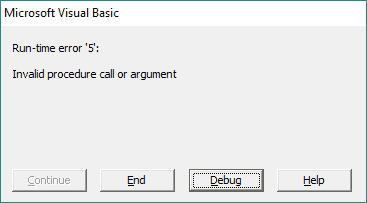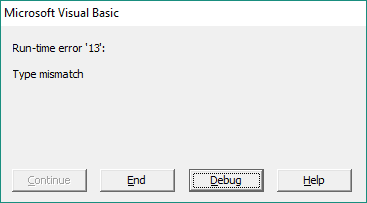Introduction | Example | Tutorial | Applications

## Introduction - Square Root in VBA

This VBA tutorial shows you how to take the square root of a number in VBA using the Sqr function. The VBA Sqr function accepts one argument - a positive number - and will return the square root of that number as a Double data type.

Let’s take a look at an example.

## Example - Square Root in VBA

### VBA Sqr Function

``````Sub VBA_Square_Root()
Dim d1 As Double
Dim d2 As Double

d1 = 144
d2 = Sqr(d1) 'Returns 12
Debug.Print d2
End Sub``````

Make powerful macros with our free VBA Developer Kit

It’s easy to copy and paste a macro like this, but it’s harder make one on your own. To help you make macros like this, we built a free VBA Developer Kit and wrote the Big Book of Excel VBA Macros full of hundreds of pre-built macros to help you master file I/O, arrays, strings and more - grab your free copy below.

I'll take a free VBA Developer Kit

## Tutorial - Square Root in VBA

### How to find the square root in VBA

The VBA Sqr function returns the square root of a number. The Sqr function accepts one argument: a positive number. Here’s the syntax for the Sqr function:

`Sqr(Number)`

In the example above, the “Number” argument is 144 so the value of d2 is 12 since 12x12=144.

### Common Errors with the Sqr function

Like I said earlier, the Sqr function accepts a positive number. Any number multiplied by itself returns a positive number so, ignoring imaginary numbers, you can’t take the square root of a negative number.

Because of that, the VBA Sqr function will produce a “Run-time error ‘5’ Invalid procedure call or argument” error when attempting to take a square root of a negative number.Similarly, you will get a “Run-time error ‘13’ Type Mismatch” error when you try to take the square root of something that isn’t a number.With that said, the Sqr function isn’t dumb. If you try to take the square root of a string that represents a number, it will properly process the string as a number and return the square root. Here’s an example to demonstrate what I mean:

``````Sub VBA_Square_Root_String()
Dim val1 As String
Dim val2 As String

val1 = "16"
val2 = Sqr(val1) 'Returns "4"
Debug.Print val2
End Sub``````

The result of applying the square root function in this example is a string with a value of “4.” If the variable val2 was instead declared as a double, the VBA square root function would be smart enough to process the string “16” as a number and return a double with a numeric value of 4.

## Application Ideas

You’ll want to use the Sqr function anytime you need to calculate the square root in VBA.

It’s wise to use the Sgn function to test for a positive number or the Abs function to return the absolute value of your number before passing it as an argument to the VBA Sqr function.

That’s all for this tutorial. When you’re ready to take your VBA to the next level, subscribe using the form below.